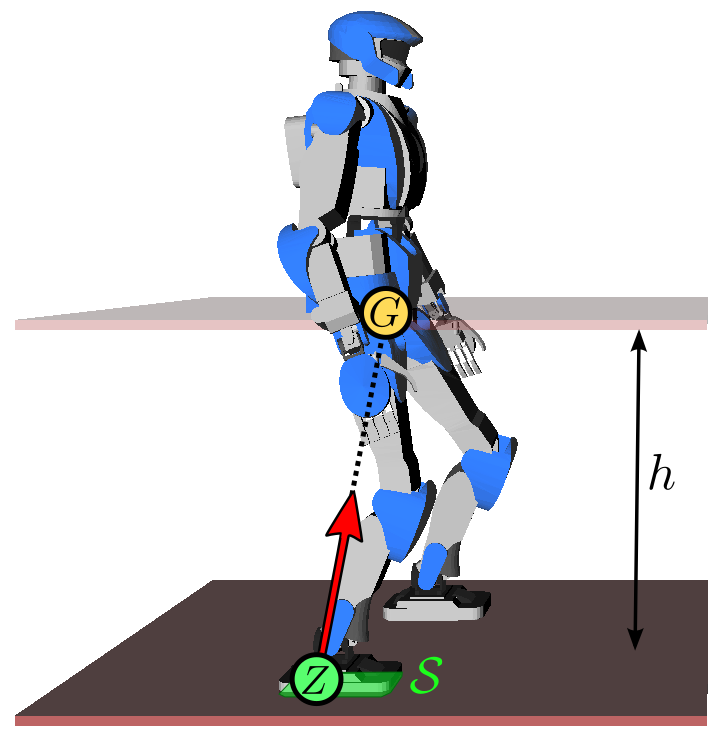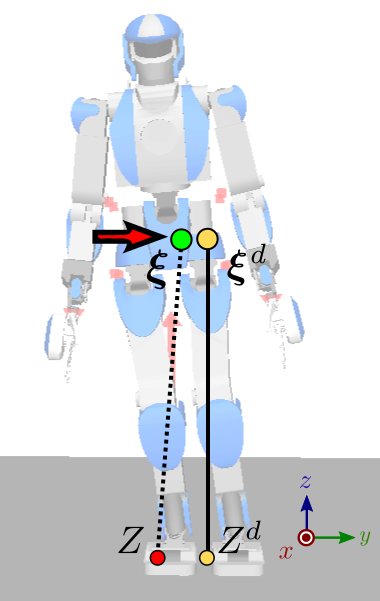# How do biped robots walk?

Walking has been realized on biped robots since the 1970s and 1980s, but a major stride in the field came in 1996 when Honda unveiled its P2 humanoid robot which would later become ASIMO. It was already capable of walking, pushing a cart and climb stairs. A key point in the design of P2 was its walking control based on feedback of the zero-tilting moment point (ZMP). Let us look at the working assumptions and components behind it.

If this is your first time reading this page, I warmly advise you watch this excellent documentary from the NHK on ASIMO. It does a very good job at explaining some of the key concepts that we are going to define more formally below.

## Linear inverted pendulum model

The common model for (fixed or mobile) robots consists of multiple rigid bodies connected by actuated joints. The general equation of motion for such a system are high-dimensional, but they can be reduced using three working assumptions:

• Assumption 1: the robot has enough joint torques to realize its motions.
• Assumption 2: there is no angular momentum around the center of mass (CoM).
• Assumption 3: the center of mass keeps a constant height.

Assumptions 2 and 3 explain why you see the Honda P2 walk with locked arms and bent knees. Under these three assumptions, the equations of motion of the walking biped are reduced to a linear model, the linear inverted pendulum:

\begin{equation*} \bfpdd_G = \omega^2 (\bfp_G - \bfp_Z) \end{equation*}

where $$\omega^2 = g / h$$, $$g$$ is the gravity constant, $$h$$ is the CoM height and $$\bfp_Z$$ is the position of the zero-tilting moment point (ZMP). The constant $$\omega$$ is called the natural frequency of the linear inverted pendulum. In this model, the robot can be seen as a point-mass concentrated at $$G$$ resting on a massless leg in contact with the ground at $$Z$$. Intuitively, the ZMP is the point where the robot applies its weight. As a consequence, this point needs to lie inside the contact surface $$\cal S$$.To walk, the robot shifts its ZMP backward, which makes its CoM accelerate forward from the above equation (intuitively, walking starts by falling forward). Meanwhile, it swings its free leg to make a new step. After the swing foot touches down on the ground, the robot shifts its ZMP to the new foothold (intuitively, it transfers its weight there), which decelerates the CoM from the equation above. Then the process repeats.

Now that we have a model, let us turn to the questions of planning and control. Walking is commonly decomposed into two sub-tasks:

• Walking pattern generation: generate a reference CoM-ZMP trajectory, assuming no disturbance and a perfect model.
• Walking stabilization: track at best this reference trajectory, using feedback control to reject disturbances and model errors.

## Walking pattern generation

The goal of walking pattern generation is to generate a CoM trajectory $$\bfp_G(t)$$ whose corresponding ZMP, derived by:

\begin{equation*} \bfp_Z = \bfp_G - \frac{\bfpdd_G}{\omega^2} \end{equation*}

lies at all times within the contact area $$\cal S$$ between the biped and its environment. If the robot is in single support (i.e. on one foot), this area corresponds to the contact surface below the sole. If the robot is in double support (two feet in contact) and a flat floor, it corresponds to the convex hull of all ground contact points. (If the ground is uneven or the robot makes other contacts (for instance leaning somewhere with its hands), the multi-contact ZMP area can be defined, but its construction is a bit more complex.)

### Linear Model Predictive Control

There are different methods to generate walking patterns. One of the most prominent ones is to formulate the problem as a numerical optimization, an approach introduced as preview control in 2003 by Kajita et al. and that has since then be extended to linear model predictive control (MPC) by Wieber et al. (also with footstep adaption and CoM height variations). This approach powers walking pattern generation for robots of the HRP series like HRP-2 and HRP-4.

### DCM Trajectory Generation

Another method (actually not incompatible with the latter) is to decompose the second-order dynamics of the LIPM into two first-order systems. Define $$\bfxi$$ as follows:

\begin{equation*} \bfxi = \bfp_G + \frac{\bfpd_G}{\omega} \end{equation*}

The dynamics of the LIPM can then be re-written as:

\begin{equation*} \begin{array}{rcl} \dot{\bfxi} & = & \omega (\bfxi - \bfp_Z) \\ \bfpd_G & = & \omega(\bfxi - \bfp_G) \end{array} \end{equation*}

The interesting thing here is that the second equation is a stable system: it has a negative feedback gain $$-\omega$$ on $$\bfp_G$$, or to say it otherwise, if the forcing term $$\bfxi$$ becomes constant then $$\bfp_G$$ will naturally converge to it. The point $$\bfxi$$ is known as the instantaneous capture point (ICP). The other equation remains unstable: the capture point $$\bfxi$$ always diverges away from the ZMP $$\bfp_Z$$, which is why $$\bfxi$$ is also called the divergent component of motion (DCM). The name instantaneous capture point comes from the fact that, if the robot would instantaneously step on this point $$\forall t \geq t_0, \bfp_Z(t) = \bfxi$$, its CoM would naturally come to a stop (be "captured") with $$\bfp_G(t) \to \bfxi$$ as $$t \to \infty$$.

As the CoM always converges to the DCM, there is no need to take care of the second equation in the dynamic decomposition above. Walking controllers become more efficient when they focus on controlling the DCM rather than both the CoM position and velocity: informally, no unnecessary control is "spent" to control the stable dynamics. Formally, controlling the DCM maximizes the basin of attraction of linear feedback controllers. Walking pattern generation can then focus on producing a trajectory $$\bfxi(t)$$ rather than $$\bfp_G(t)$$. Since the equation $$\dot{\bfxi} = \omega (\bfxi - \bfp_Z)$$ is linear, this can be done using geometric or analytic solutions. These DCM trajectory generation methods power walking pattern generation for ASIMO, IHMC's Atlas or TORO humanoid robots.

Now that we have a reference walking pattern, we want to make the real robot execute it. Simple open-loop playback won't work here, as we saw that the dynamics of walking are naturally diverging (walking is a "controlled fall"). We will therefore add feedback to it.

## Walking stabilization

In 1996, the Honda P2 introduced two key developments: on the hardware side, a rubber bush added between the ankle and the foot sole to absorb impacts and enable compliant control of the ground reaction force, and on the software side, feedback control of the ZMP. Using the terminology from ASIMO's balance control report, this feedback law can be expressed using the DCM

\begin{equation*} \dot{\bfxi} = \dot{\bfxi}^d + k_\xi (\bfxi^d - \bfxi) \end{equation*}

where $$\bfxi^d$$ is the desired DCM from the walking pattern. Given the equation above where $$\dot{\bfxi} = \omega (\bfxi - \bfp_Z)$$, this feedback law can be rewritten equivalently in terms of the ZMP:

\begin{equation*} \bfp_Z = \bfp_Z^d + k_z (\bfxi - \bfxi^d) \end{equation*}

where $$k_Z = 1 + k_\xi / \omega$$, $$\bfp_Z^d$$ is the desired ZMP from the walking pattern and $$\bfp_Z$$ is the ZMP controlled by the robot using foot force control. For position-controlled robots such as HRP-2 or HRP-4, foot force control can be realized by damping control of ankle joints, see for instance Section III.D of the reference report on HRP-4C's walking stabilizer. This report is in itself an excellent read and I warmly encourage you to read it if you want to learn more about walking stabilization: every section of it is meaningful.So, what happens with this control law? Imagine for instance that, while playing back the walking pattern, the robot starts tilting to the right for some reason (umodeled dynamics, tilted ground, ...) As a consequence, the lateral coordinate $$\xi_y$$ of the DCM will become lower than $$\xi^d$$. As a consequence of the feedback law above, the ZMP will then shift toward $$y_Z < y_Z^d$$, generating a positive velocity

\begin{equation*} \dot{\xi}_y = \omega (\xi_y - y_Z) = \dot{\xi}^d_y + k_\xi (\xi_y^d - \xi_y) \end{equation*}

on the DCM (red arrow on the figure to the right) that brings it closer to the desired one. Hand-wavingly, the robot is tilting its right foot to the right in order to push itself back to its left.

## To go further

Is that it? Well, yes, at least for a global overview. Follow the links inlined in the discussion above for specifics on each part. The main point I didn't mention above is called state observation: in this instance, how to estimate the CoM position and velocity from sensory measurements. Here are some other pointers on walking control itself:

There are other families of walking control methods that do not (at least explicitly) rely on ZMP feedback, notably passive walkers and hybrid zero dynamics which powers the DURUS biped.

Pages of this website are under the CC-BY 4.0 license.